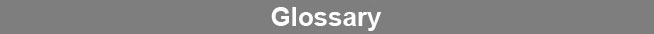Select Page### Symmetrical Dilution Curve

A symmetrical dilution curve is one that yields a sigmoidal curve with both asymptotes elongated equally when the response of each dilution is plotted against the logarithm of its respective concentration. The inflection point of a symmetrical dilution curve, where the mirror image of one half of the curve is superimposable on the other half, is the midpoint of the curve.www.brendan.com